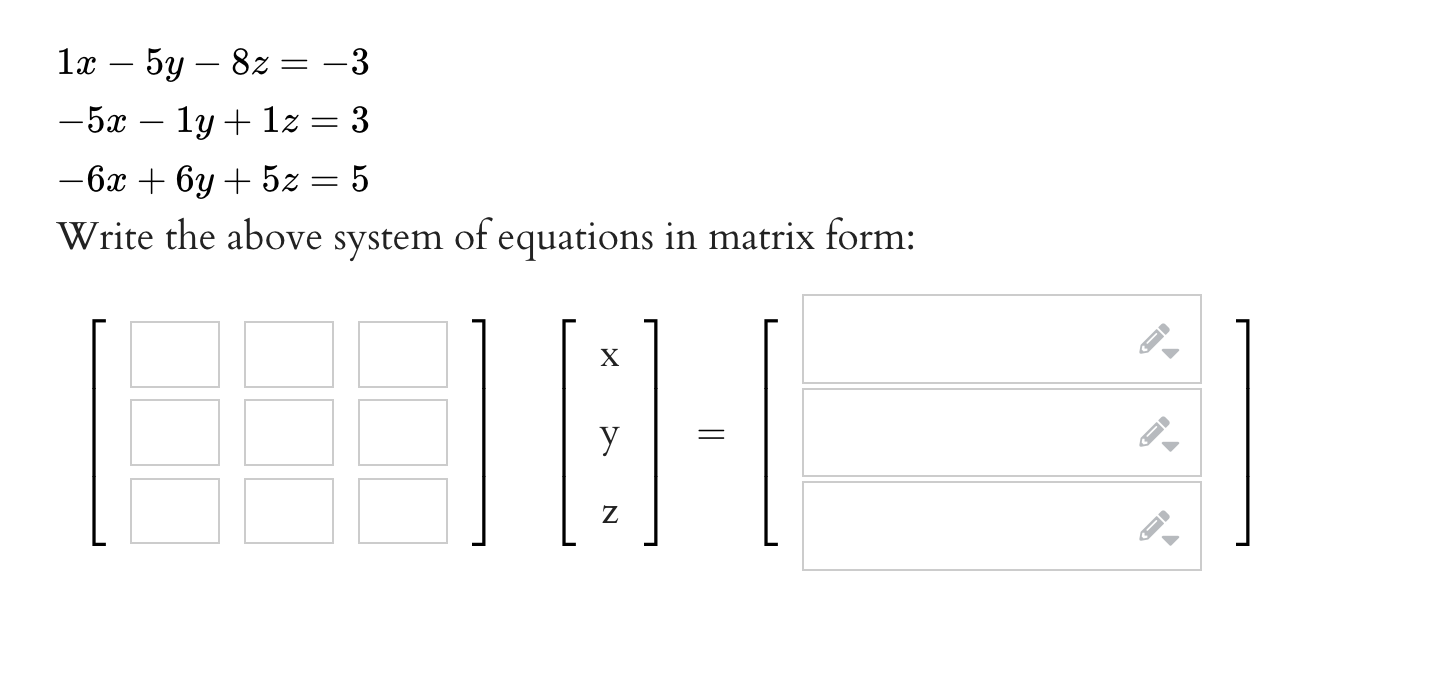Home / Expert Answers / Algebra / begin-array-l-1-x-5-y-8-z-3-5-x-1-y-1-z-3-6-x-6-y-5-z-5-end-array-write-the-ab-pa291

# (Solved): $\begin{array}{l} 1 x-5 y-8 z=-3 \\ -5 x-1 y+1 z=3 \\ -6 x+6 y+5 z=5 \end{array}$ Write the ab ...$\begin{array}{l} 1 x-5 y-8 z=-3 \\ -5 x-1 y+1 z=3 \\ -6 x+6 y+5 z=5 \end{array}$ Write the above system of equations in matrix form: $\left[\begin{array}{l} \mathrm{x} \\ \mathrm{y} \\ \mathrm{z} \end{array}\right]=\left[\begin{array}{l} 1 \end{array}\right]$

We have an Answer from Expert